##### Pre-Calculus Workbook For Dummies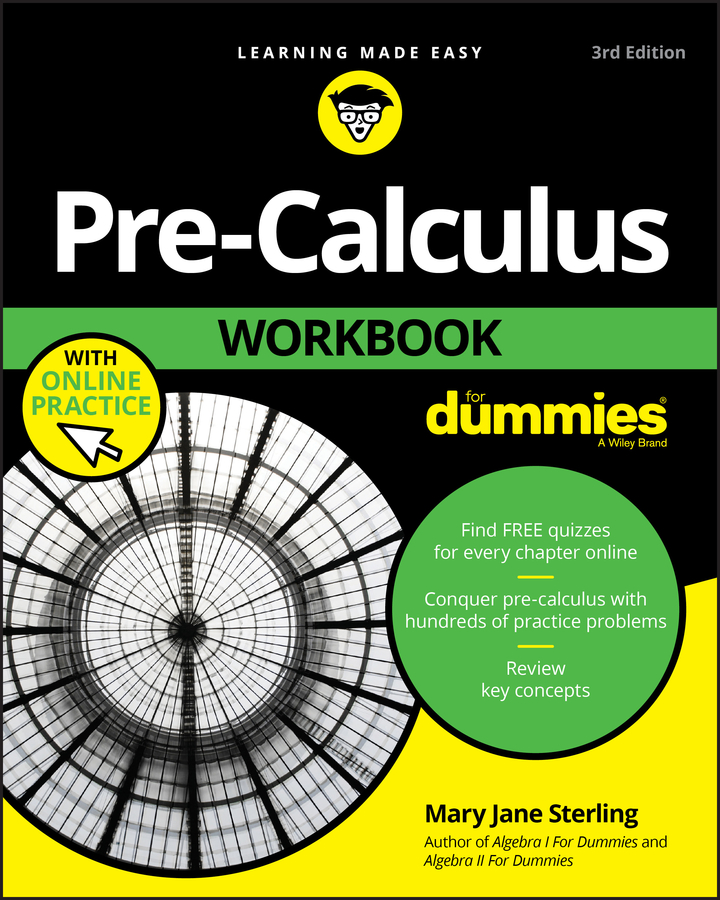You use a double-angle formula to find the trig value of twice an angle. Sometimes you know the original angle; sometimes you don't. Working with double-angle formulas comes in handy when you're given the sine of an angle and need to find the exact trig value of twice that angle without knowing the measure of the original angle.

Note: If you know the original angle in question, finding the sine of twice that angle is easy; you can look it up on the unit circle (shown in the figure) or use your calculator to find the answer.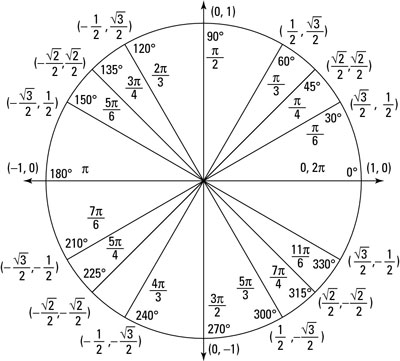The whole unit circle

However, if you don't have the measure of the original angle and you must find the exact value of twice that angle, the process isn't as simple. Read on!

To fully understand and be able to stow away the double-angle formula for sine, you should first understand where it comes from. (The double-angle formulas for sine, cosine, and tangent are extremely different from one another, although they can all be derived by using the sum formulas.)

1. To find sin 2x, you must realize that it's the same as sin(x + x).

2. Use the sum formula for sine,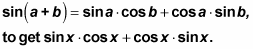3. Simplify to get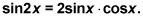This formula is called the double-angle formula for sine. If you're given an equation with more than one trig function and asked to solve for the angle, your best bet is to express the equation in terms of one trig function only. You often can achieve this by using the double-angle formula.

To solve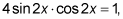notice that it doesn't equal 0, so you can't factor it. Even if you subtract 1 from both sides to get 0, it still can't be factored. So there's no solution, right? Not quite. You have to check the identities first. The double-angle formula, for instance, says that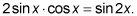You can rewrite some things here:

1. List the given information.

You have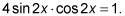2. Rewrite the equation to find a possible identity.

You go with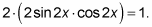3. Apply the correct formula.

The double-angle formula for sine gives you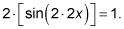4. Simplify the equation and isolate the trig function.

Break it down to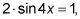which becomes sin 4x = 1/2.

5. Find all the solutions for the trig equation.

This step gives you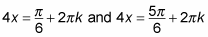where k is an integer. Note that there are two sets of solutions because sin (pi/6) and sin (5pi/6) both equal ½. You use the notation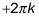to represent the fact that the sine function has a period of 2pi, meaning it repeats itself every 2pi units. Then you can divide everything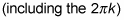by 4, which gives you the solutions: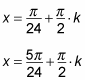These solutions are the general ones, but sometime you may have to use this information to get to a solution on an interval.

Finding the solutions on an interval is a curveball thrown at you in pre-calculus. For this problem, you can find a total of eight angles on the interval [0, 2pi). Because a coefficient was in front of the variable, you're left with, in this case, four times as many solutions, and you must state them all. You have to find the common denominator to add the fractions.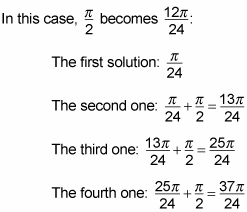Doing this one more time gets you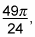which is not in the interval [0, 2pi). Meanwhile,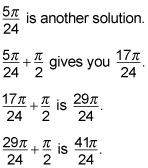You stop there, because adding pi/2 once more would get you a solution that is not in the interval [0, 2pi).